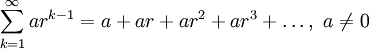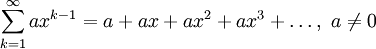# A Motivating Problem for Power Series

Previous: Power Series

Next: The Power Series

Earlier in the Infinite Series Module, we introduced the concept of a geometric series,We assumed that r was a constant. But what if r were not a constant, and instead was a variable?

Let's replace our r with x in the above summation. We now obtainFrom this simple substitution, we have created a function, and can ask two important questions related to this result.

## Key Questions for This Lesson

From our simple construction, we can ask:

1. for what values of x does our summation yield a finite result?
2. is there a more general representation of our summation?

The first question will take us to the idea of a radius of convergence, and the second question to the concept of a power series.

Previous: Power Series

Next: The Power Series